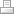Upcoming shows/vending:

Know of a show I should go to? Tell me!

# Hands-free Garbage Can

Original code for 4-pin ultrasonic sensor here. My code below adds the servo functions:

/*Auto Garbage Can Lid Openering Thing
with HC-SR04 Sensor

*/
const int servo = 11;
const int ledpin = 13;
const int trigPin = 2;
const int echoPin = 4;
int potpin = A0;

int rotations = 0;

const int clockwise = 1700;
const int counterclockwise = 1300;

void setup() {
// initialize serial communication:
Serial.begin(9600);
pinMode(ledpin, OUTPUT);
digitalWrite(ledpin,LOW);
}

void loop()
{

long duration, inches, cm;
rotations = map (rotations,0, 1023, 10, 400);

// The sensor is triggered by a HIGH pulse of 10 or more microseconds.
// Give a short LOW pulse beforehand to ensure a clean HIGH pulse:
pinMode(trigPin, OUTPUT);
digitalWrite(trigPin, LOW);
delayMicroseconds(2);
digitalWrite(trigPin, HIGH);
delayMicroseconds(10);
digitalWrite(trigPin, LOW);

// Read the signal from the sensor: a HIGH pulse whose
// duration is the time (in microseconds) from the sending
// of the ping to the reception of its echo off of an object.
pinMode(echoPin, INPUT);
duration = pulseIn(echoPin, HIGH);

// convert the time into a distance
inches = microsecondsToInches(duration);
cm = microsecondsToCentimeters(duration);

//Serial.print(inches); Serial.print("in, ");
//Serial.print(cm); Serial.print("cm");  Serial.println();
Serial.print(rotations); Serial.println();

if (inches < 5) {
digitalWrite(ledpin,HIGH);
for(int i=0; i<rotations; i++)
{
digitalWrite(servo,HIGH);
delayMicroseconds(clockwise);
digitalWrite(servo,LOW);
delay(18.5); // 18.5ms
//delay(50);
}
delay(3000);
for(int i=0; i<rotations; i++)
{
digitalWrite(servo,HIGH);
delayMicroseconds(counterclockwise);
digitalWrite(servo,LOW);
delay(18.5); // 18.5ms

}}

if (inches > 5) {
digitalWrite(ledpin,LOW);}

delay(100);
}

long microsecondsToInches(long microseconds)
{
// According to Parallax's datasheet for the PING))), there are
// 73.746 microseconds per inch (i.e. sound travels at 1130 feet per
// second).  This gives the distance travelled by the ping, outbound
// and return, so we divide by 2 to get the distance of the obstacle.
// See: http://www.parallax.com/dl/docs/prod/acc/28015-PING-v1.3.pdf
return microseconds / 74 / 2;
}

long microsecondsToCentimeters(long microseconds)
{
// The speed of sound is 340 m/s or 29 microseconds per centimeter.
// The ping travels out and back, so to find the distance of the
// object we take half of the distance travelled.
return microseconds / 29 / 2;
}Print | Sitemap